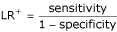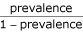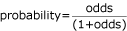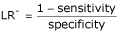# Statistics review 13: Receiver operating characteristic curves

## Abstract

This review introduces some commonly used methods for assessing the performance of a diagnostic test. The sensitivity, specificity and likelihood ratio of a test are discussed. The uses of the receiver operating characteristic curve and the area under the curve are explained.

## Introduction

A simple diagnostic test for a particular disease or outcome classifies patients into two groups: those with the outcome and those without. A test is assessed by its ability to diagnose the outcome correctly, whether this is positive or negative. If the actual outcome is not evident then it may be supplied by the 'gold standard' test. The data given in Table 1 provide an example in which the outcome is death or survival. The patients were attending an accident and emergency unit and the venous blood analysis for the metabolic marker lactate was used in the early identification of those patients at risk for death. Patients with lactate levels greater than 1.5 mmol/l were considered to be at risk. In general, the results of a diagnostic test may be presented as shown in Table 2.

## Sensitivity and specificity

The sensitivity of a diagnostic test is the proportion of patients for whom the outcome is positive that are correctly identified by the test. The specificity is the proportion of patients for whom the outcome is negative that are correctly identified by the test.

For the data given in Table 1 the sensitivity of the test using lactate level above 1.5 mmol/l as an indicator of mortality is 81/126 = 0.64, and the specificity is 674/1265 = 0.53. Therefore, 64% of patients in this sample who died and 53% who survived were correctly identified by this test. Because both of these measures are simple proportions, their confidence intervals can be calculated as described in Statistics review 8 . The 95% confidence interval for sensitivity is 56–73% and that for specificity is 51–56%.

Generally, both the sensitivity and specificity of a test need to be known in order to assess its usefulness for a diagnosis. A discriminating test would have sensitivity and specificity close to 100%. However, a test with high sensitivity may have low specificity and vice versa. The decision to make use of a diagnostic test will also depend on whether a treatment exists should the result of the test be positive, the cost of such a treatment, and whether the treatment is detrimental in cases in which the result is a false positive.

## Positive and negative predictive values

The positive predictive value (PPV) of a test is the probability that a patient has a positive outcome given that they have a positive test result. This is in contrast to sensitivity, which is the probability that a patient has a positive test result given that they have a positive outcome. Similarly, the negative predictive value (NPV) is the probability that a patient has a negative outcome given that they have a negative test result, in contrast to specificity, which is the probability that a patient has a negative test result given that they have a negative outcome.

For the data in Table 1 the PPV of the test using lactate level above 1.5 mmol/l as an indicator of mortality is 81/672 = 0.12, and the NPV is 674/719 = 0.94. Therefore, 12% of patients in the sample whose test results were positive actually died and 94% whose test results were negative survived. The 95% confidence interval for PPV is 10–15% and that for NPV is 92–96%.

Sensitivity and specificity are characteristics of a test and are not affected by the prevalence of the disease. However, although the PPV and NPV give a direct assessment of the usefulness of the test, they are affected by the prevalence of the disease. For example, Table 3 uses the same sensitivity, specificity and sample size as for the data in Table 1, but the prevalence (proportion of deaths) has been changed from 126/1391 = 9% to 600/1391 = 43%. The PPV and NPV are now 386/756 = 0.51 and 421/635 = 0.66, respectively. The increase in prevalence has led to an increase in PPV and a decrease in NPV. When the prevalence is low the PPV will be low, irrespective of the sensitivity and specificity of the test. A higher prevalence will always result in a raised PPV and a lowered NPV.

## Likelihood ratios

Sensitivity and specificity are usefully combined in likelihood ratios. The likelihood ratio of a positive test result (LR+) is the ratio of the probability of a positive test result if the outcome is positive (true positive) to the probability of a positive test result if the outcome is negative (false positive). It can be expressed as follows:LR+ represents the increase in odds favouring the outcome given a positive test result. For the data in Table 1, LR+ is 0.64/(1 - 0.53) = 1.36. This indicates that a positive result is 1.36 times as likely for a patient who died as for one who survived.

The pre-test probability of a positive outcome is the prevalence of the outcome. The pre-test odds  can be used to calculate the post-test probability of outcome and are given by:Applying Bayes' theorem , we have:

Post-test odds for the outcome given a positive test result = pre-test odds × LR+

For the data given in Table 1, the prevalence of death = 126/1391 = 0.09 and the pre-test odds of death = 0.09/(1 - 0.09) = 0.099. Therefore:

Post-test odds of death given a positive test result = 0.099 × 1.36 = 0.135

For a simpler interpretation, these odds can be converted to a probability using the following:For the data in Table 1 this gives a probability = 0.135/(1 + 0.135) = 0.12. This is the probability of death given a positive test result (i.e. the PPV).

Similarly, we can define LR- as the ratio of the probability of a negative test result if the outcome is positive to the probability of a negative test result if the outcome is negative. It can be expressed as follows:LR- represents the increase in odds favouring the outcome given a negative test result. For the data given in Table 1, LR- is (1 - 0.64)/0.53 = 0.68. This indicates that a negative result is 0.68 times as likely for a patient who died as for one who survived. Applying Bayes' theorem, we have the following:

Post-test odds for the outcome given a negative test result = pre-test odds × LR-

For the data in Table 1:

Post-test odds of death given a negative test result = 0.099 × 0.68 = 0.067

Converting these odds to a probability gives 0.067/(1 + 0.067) = 0.06. This is the probability of death given a negative test result (i.e. 1 - NPV). Therefore, NPV = 1 - 0.06 = 0.94, as shown above.

A high likelihood ratio for a positive result or a low likelihood ratio for a negative result (close to zero) indicates that a test is useful. As previously stated, a greater prevalence will raise the probability of a positive outcome given either a positive or a negative test result.

## Youden's index

When a diagnostic test is based on a continuous measurement, a range of different decision thresholds or cutoff values may be investigated in order to decide which value should be used to discriminate between patients according to outcome. The data given in Table 1 used lactate measurement with a cut-off of 1.5 mmol/l. Table 4 shows the numbers of patients who died or survived classified according to a range of cut-off values. The sensitivity and specificity have been calculated for each of these cut-off values and these are also shown in Table 4. For example, the sensitivity of a test using a cut-off of 2 mmol/l is calculated as 58/126 = 0.46, and the specificity as (1265 - 329)/1265 = 0.74.

It is desirable to choose a test that has high values for both sensitivity and specificity. In practice, the sensitivity and specificity may not be regarded as equally important. For example, a false-negative finding may be more critical than a false-positive one, in which case a cut-off with a relatively high specificity would be chosen. However, if no judgement is made between the two, then Youden's index (J) may be used to choose an appropriate cut-off:

J = sensitivity + specificity - 1

The maximum value J can attain is 1, when the test is perfect, and the minimum value is usually 0, when the test has no diagnostic value. From Table 4, the best cut-off value for lactate using Youden's index is 2 mmol/l, with J = 0.20

## Receiver operating characteristic curve and area under the curve

When the cut-off value for a continuous diagnostic variable is increased (assuming that larger values indicate an increased chance of a positive outcome), the proportions of both true and false positives decreases. These proportions are the sensitivity and 1 – specificity, respectively. A graph of sensitivity against 1 – specificity is called a receiver operating characteristic (ROC) curve. Figure 1 shows the ROC curve for lactate using the cut-off values given in Table 4. The preferred method is to join the points by straight lines but it is possible to fit a smooth curve from a parametric model.

A perfect test would have sensitivity and specificity both equal to 1. If a cut-off value existed to produce such a test, then the sensitivity would be 1 for any non-zero values of 1 – specificity. The ROC curve would start at the origin (0,0), go vertically up the y-axis to (0,1) and then horizontally across to (1,1). A good test would be somewhere close to this ideal.

If a variable has no diagnostic capability, then a test based on that variable would be equally likely to produce a false positive or a true positive:

Sensitivity = 1 – specificity, or

Sensitivity + specificity = 1

This equality is represented by a diagonal line from (0,0) to (1,1) on the graph of the ROC curve, as shown in Fig. 1 (dashed line).

Figure 1 suggests that lactate does not provide a very good indication of mortality but that it is better than a random guess.

The performance of a diagnostic variable can be quantified by calculating the area under the ROC curve (AUROC). The ideal test would have an AUROC of 1, whereas a random guess would have an AUROC of 0.5. The AUROC can be calculated as a sum of the areas of trapeziums. For example, in Fig. 1 the area under the curve between points (0.26,0.46) and (0.47,0.53) is given by (0.47 - 0.26) × (0.46 + 0.53)/2 = 0.10 or, in other words, the difference between the x-values multiplied by half the sum of the y-values. Alternatively, a statistical package can be used and the calculations based on cut-off values taking each of the full range of data values. Figure 2 shows the ROC curve and Table 5 shows that the AUROC for the lactate data is 0.64. This is interpreted as the probability that a patient who dies has a lactate value greater than that for a patient who survives.

Table 5 also includes the results of a hypothesis test of whether the AUROC is greater than 0.5, that is, whether using lactate to diagnose mortality is better than chance alone. The P value is less than 0.001 and the confidence interval for AUROC is 0.59–0.69, suggesting that lactate level does help to predict mortality. This procedure is equivalent to testing whether the lactate levels for those who died are generally higher than for those who survived, and therefore the Mann–Whitney test  can be used, resulting in the same P value.

## Choosing between diagnostic tests

The ability of two continuous variables to diagnose an outcome can be compared using ROC curves and their AUROCs. For example, Fig. 3 and Table 6 show the ROC curve and AUROC for urea in addition to those for lactate. The AUROC for urea is greater than that for lactate, suggesting that urea may provide a better predictive test for mortality. A formal test would be necessary to show whether the difference is significant. Such tests are possible but not readily available in statistical packages [4, 5]. In comparisons of this sort the differences in shape of the curves may be important. In this example it can be seen in Fig. 3 that, for very low levels of sensitivity, lactate has a higher level of specificity than urea. If a cut-off is selected for a high level of specificity, then lactate may be more discriminating.

## Assumptions and limitations

Sensitivity and specificity may not be invariant for a diagnostic test but may depend on characteristics of the population, for example age profile or severity of disease.

The decision to use a diagnostic test depends not only on the ROC analysis but also on the ultimate benefit to the patient. The prevalence of the outcome, which is the pre-test probability, must also be known.

Generally, there is a trade-off between sensitivity and specificity, and the practitioner must make a decision based on their relative importance.

## Conclusion

ROC analysis provides a useful means to assess the diagnostic accuracy of a test and to compare the performance of more than one test for the same outcome. However, the usefulness of the test must be considered in the light of the clinical circumstances.

## Abbreviations

AUROC:

area under the receiver operating characteristic curve

PLR:

positive likelihood ratio

NLR:

negative likelihood ratio

NPV:

negative predictive value

PPV:

positive predictive value

ROC:

## References

1. Bewick V, Cheek L, Ball J: Statistics review 8: Qualitative data – tests of association. Crit Care 2004, 8: 46-53. 10.1186/cc2428

2. Petrie A, Sabin C: Medical Statistics at a Glance. Oxford, UK: Blackwell; 2000.

3. Whitley E, Ball J: Statistics review 6: Nonparametric methods. Crit Care 2002, 6: 509-513. 10.1186/cc1820

4. Campbell MJ, Machin D: Medical Statistics: A Commonsense Approach. 3rd edition. Chichester, UK: Wiley; 1999.

5. Hanley JA, McNeil BJ: A method of comparing the areas under receiver operating characteristic curves derived from the same cases. Radiology 1983, 148: 839-843.

## Author information

Authors

### Corresponding author

Correspondence to Viv Bewick.

### Competing interests

The author(s) declare that they have no competing interests.

## Authors’ original submitted files for images

Below are the links to the authors’ original submitted files for images.

## Rights and permissions

Reprints and Permissions

Bewick, V., Cheek, L. & Ball, J. Statistics review 13: Receiver operating characteristic curves. Crit Care 8, 508 (2004). https://doi.org/10.1186/cc3000

• Published:

• DOI: https://doi.org/10.1186/cc3000

### Keywords

• AUROC
• negative likelihood ratio
• negative predictive value
• positive likelihood ratio
• positive predictive value
• ROC curve
• sensitivity
• specificity